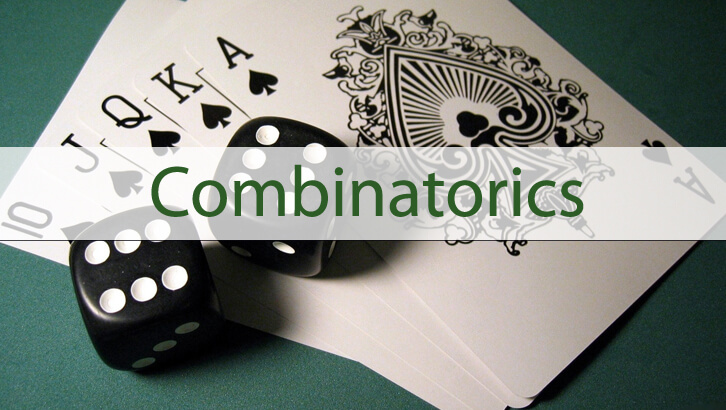# Few Questions on Number Theory and Counting (combined)

CAT has been consistently asking questions combining basic number theory and counting. So, it is probably good practice to have a go at these.

1. From the digits 2,3,4,5,6 and 7, how many 5-digit numbers can be formed that have distinct digits and are multiples of 12?

2. All numbers from 1 to 200 (in decimal system) are written in base 6 and base 7 systems. How many of the numbers will have a non-zero units digit in both base 6 and base 7 notations?

3. All numbers from 1 to 150 (in decimal system) are written in base 6 notation. How many of these will not contain any zero?

4. How many factors of 1080 are perfect squares?

Solutions to these questions can be found here.

## 3 comments

1.Sriram says:

qn3: 125?
qn2,3: multiples of 6, 7 will have 0s.
qn1: 2,3,4,5,7;
2,4,5,6,7 possible cos sum upto 3(requirement for 12 multiple) and from tat numbers with last2 dgits as mult of 4 are only ok. 5 nos?

2.Sriram says:

qn.4 – 2cube*3cube*5, so 2square, 3 square and 2*3 square
4 are perfect squares cos one should not forget 1? 🙂

3.Vivek says:

td